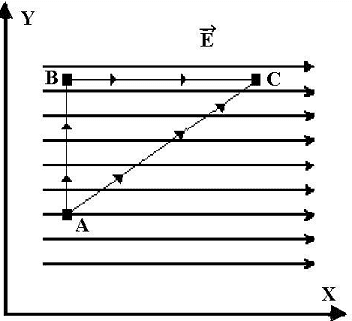# Electric potential and electric potential energy concept questions

j.frank52
Hello. This is my first post! Thanks in advance for any help given.

## Homework Statement

A constant electric field is in the positive X direction as shown in the diagram below. Also shown are two paths through which a charge can move from point A to point C. In the first path path the charge moves parallel to the Y-axis to B and then parallel to the X-axis to C. In the second path, the charge moves directly from A to C.Select greater than, less than or equal to for the following statements.

1. The electric potential at point A is ___________ the electric potential at point B.

2. The work done on a -17.0 C charge moved along the first path is __________ the work done on a -17.0 C charge moved along the second path.

3. The electric potential energy of a -17.0 C charge at point B is ___________ the electric potential energy of a -17.0 C charge at point C.

W=Fd
V=U/q

## The Attempt at a Solution

1. For this I put equal to because the A and B are on the same equipotential surface.

2. For this I put equal to because work is path independent.

3. For this one I'm unsure how to explain in terms of equations or concepts. I have multiple tries to guess and get the right answer, but I wish to know of how I can actually get to the right choice for this one. Any suggestions or hints?

## Answers and Replies

Homework Helper
3. For this one I'm unsure how to explain in terms of equations or concepts. I have multiple tries to guess and get the right answer, but I wish to know of how I can actually get to the right choice for this one. Any suggestions or hints?
Is there a potential difference between B and C? Which direction is the force on a negative charge?

AM

Homework Helper
What is the definition of electric potential? How are work of the electric field and potential difference related?

ehild

j.frank52
Thanks!